# Ranking Rows of Pandas DataFrame

To rank the rows of Pandas DataFrame we can use the `DataFrame.rank() `method which returns a rank of every respective index of a series passed. The rank is returned on the basis of position after sorting.

Example #1 :

Here we will create a DataFrame of movies and rank them based on their ratings.

 `# import the required packages  ` `import` `pandas as pd  ` ` `  `# Define the dictionary for converting to dataframe  ` `movies ``=` `{``'Name'``: [``'The Godfather'``, ``'Bird Box'``, ``'Fight Club'``], ` `         ``'Year'``: [``'1972'``, ``'2018'``, ``'1999'``], ` `         ``'Rating'``: [``'9.2'``, ``'6.8'``, ``'8.8'``]} ` ` `  `df ``=` `pd.DataFrame(movies) ` `print``(df) `

Output: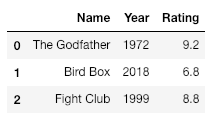`# Create a column Rating_Rank which contains ` `# the rank of each movie based on rating ` `df[``'Rating_Rank'``] ``=` `df[``'Rating'``].rank(ascending ``=` `1``) ` ` `  `# Set the index to newly created column, Rating_Rank ` `df ``=` `df.set_index(``'Rating_Rank'``) ` `print``(df) `

Output: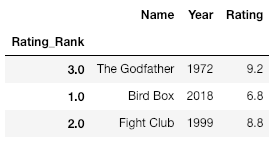`# Sort the dataFrame based on the index ` `df ``=` `df.sort_index() ` `print``(df) `

Output: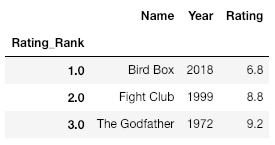Example #2
Let’s take an example of marks scored by 4 students. We will rank the students based on the highest mark they have scored.

 `# Create a dictionary with student details ` `student_details ``=` `{``'Name'``:[``'Raj'``, ``'Raj'``, ``'Raj'``, ``'Aravind'``, ``'Aravind'``, ``'Aravind'``, ` `                             ``'John'``, ``'John'``, ``'John'``, ``'Arjun'``, ``'Arjun'``, ``'Arjun'``], ` `                   ``'Subject'``:[``'Maths'``, ``'Physics'``, ``'Chemistry'``, ``'Maths'``, ``'Physics'``, ` `                             ``'Chemistry'``, ``'Maths'``, ``'Physics'``, ``'Chemistry'``, ``'Maths'``, ` `                                                           ``'Physics'``, ``'Chemistry'``], ` `                   ``'Marks'``:[``80``, ``90``, ``75``, ``60``, ``40``, ``60``, ``80``, ``55``, ``100``, ``90``, ``75``, ``70``] ` `               ``} ` ` `  `# Convert dictionary to a DataFrame ` `df ``=` `pd.DataFrame(student_details) ` `print``(df) `

Output: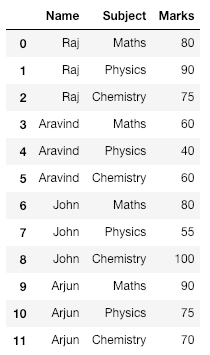`# Create a new column with Marks  ` `# ranked in descending order ` `df[``'Mark_Rank'``] ``=` `df[``'Marks'``].rank(ascending ``=` `0``) ` ` `  `# Set index to newly created column  ` `df ``=` `df.set_index(``'Mark_Rank'``) ` `print``(df) `

Output: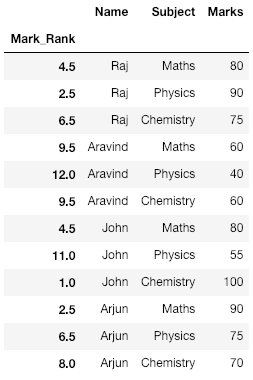`# Sort the DataFrame based on the index  ` `df ``=` `df.sort_index() ` ` `  `print``(df) `

Output: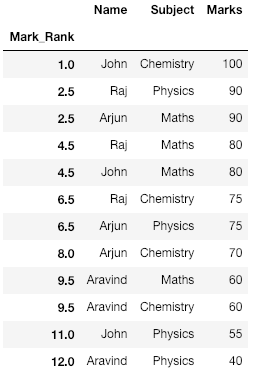Explanation:
Notice here that we have Raj and Arjun getting 90 marks each and hence they get ranked 2.5 (average of 2nd and 3rd rank i.e the two ranks they share). This can be seen for other marks in the table as well.

My Personal Notes arrow_drop_upCheck out this Author's contributed articles.

If you like GeeksforGeeks and would like to contribute, you can also write an article using contribute.geeksforgeeks.org or mail your article to contribute@geeksforgeeks.org. See your article appearing on the GeeksforGeeks main page and help other Geeks.

Please Improve this article if you find anything incorrect by clicking on the "Improve Article" button below.

Article Tags :

1

Please write to us at contribute@geeksforgeeks.org to report any issue with the above content.